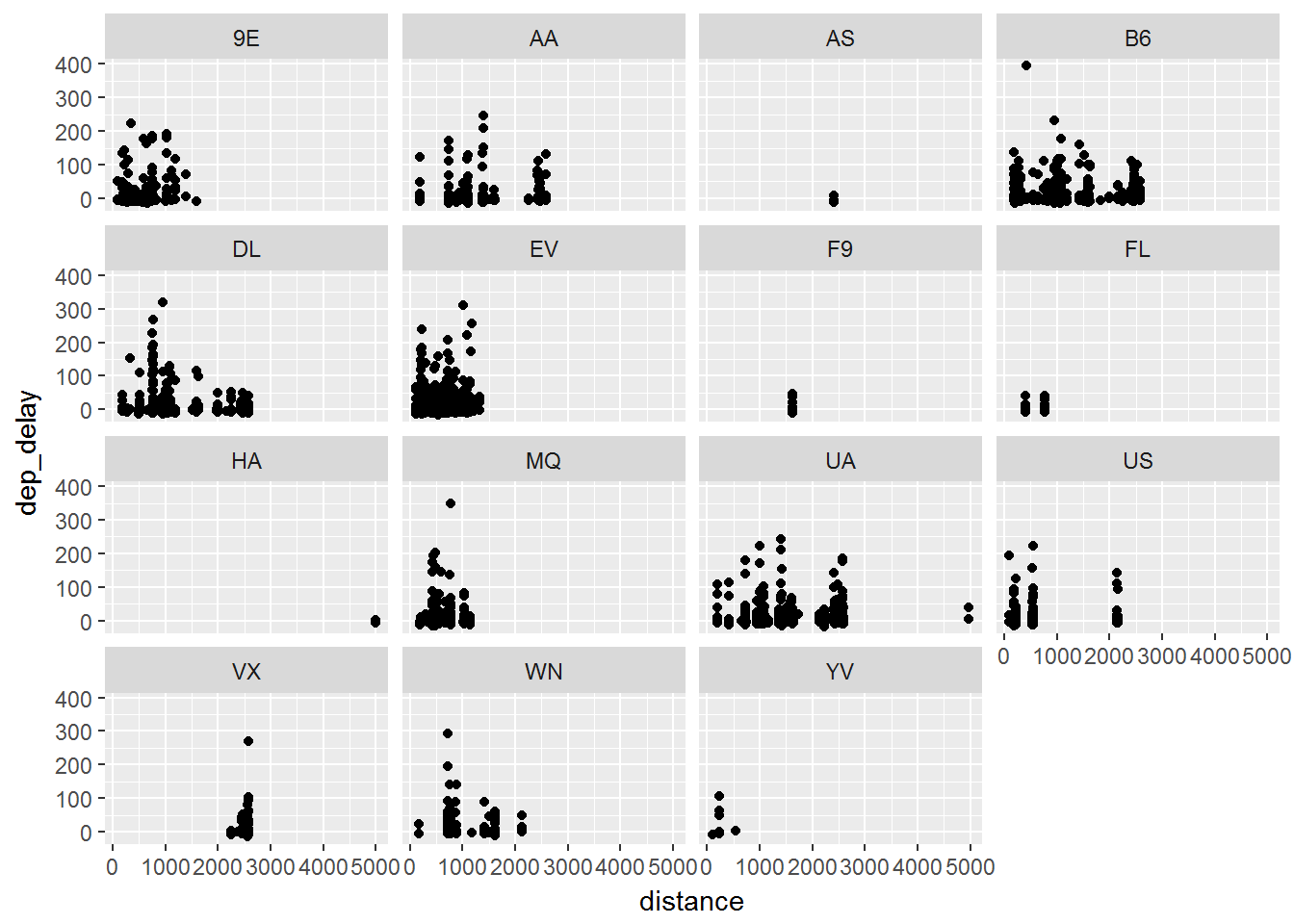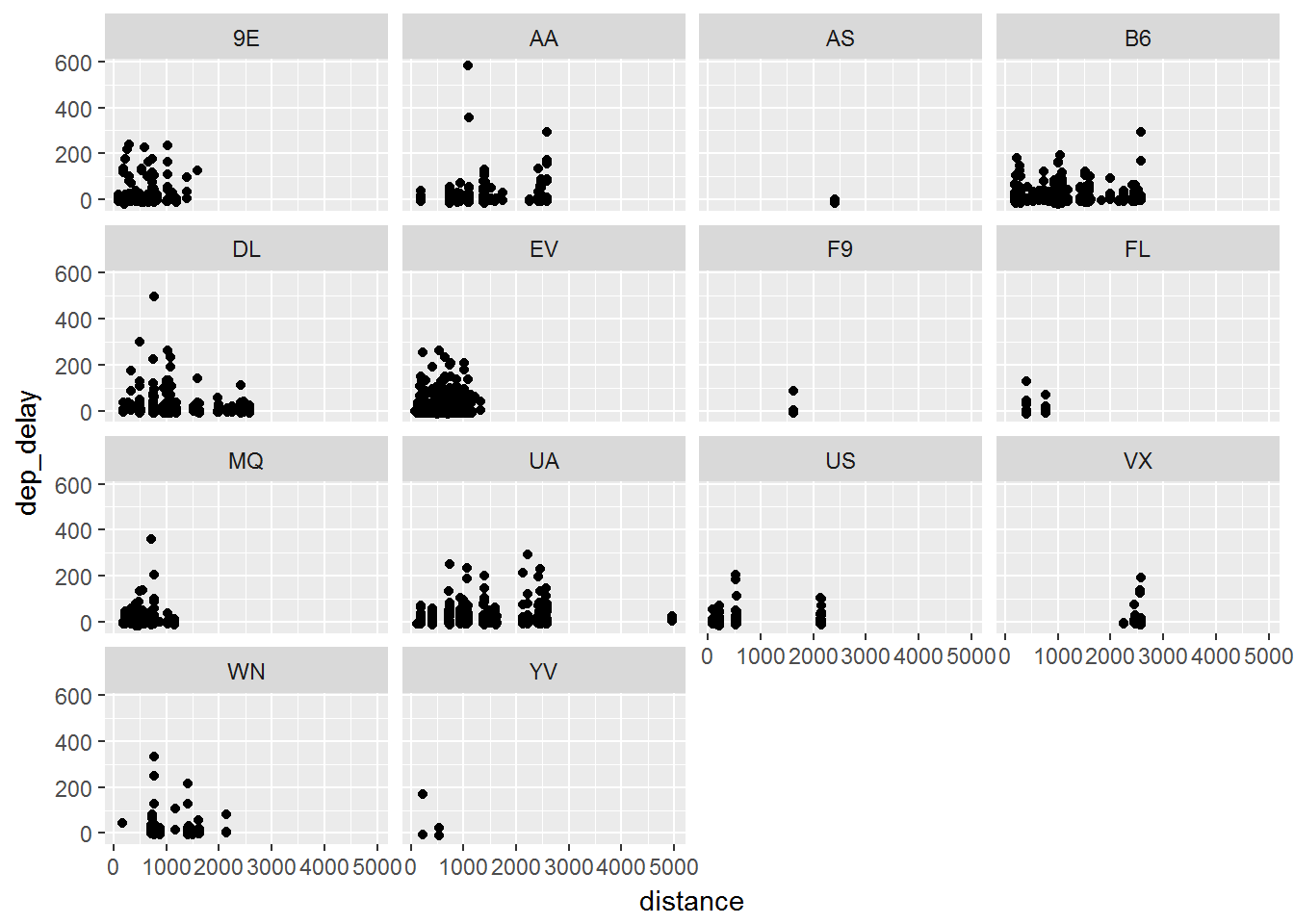# Faceting

Facetting is an excellent way to look at categorical data. This is where we split up the graphs and create a graph for each category. We will learn about two basic functions:

`facet_wrap`

`facet_grid`

## `facet_wrap`

We first will consider a `facet_wrap`:

``````data = flights %>% sample_frac(.01)

ggplot(data, aes(x=distance, y= dep_delay)) +
geom_point() +
facet_wrap(~carrier)``````

Notice that we are still working with the distance versus delay. We start out with our original scatter plot. Then we add yet another `layer`. This layer is the `facet_wrap()` where we wrap it based on carrier. Below you can see the results for this.Given the hard to read x-axis, it may be worthwhile to scale the distance differently to better see what happens.

## `facet_grid`

We then will note a similar effect when we use `facet_grid()`:

``````data = flights %>% sample_frac(.01)

ggplot(data, aes(x=distance, y= dep_delay)) +
geom_point() +
facet_grid(~carrier)``````

This is where the language of graphs really helps. We first take the data and group it based on distance and departure delay. We state to place these as points on a graph. Finally we use the `facet_grid()` to take that plot and split it by the carrier. Each time you add a layer you can accomplish a little more towards your goal.So far we have been focusing on scatter plots. As we continue to move through this section we will note that there are many other `geom` functions that can be used:
`geom_smooth` fits a smoothing line in data
`geom_boxplot` box and whisker plot of data
`geom_histogram` and `geom_freqpoly` distribution graphs
`geom_bar` distribution of categorical data
`geom_path` and `geom_line` lines between data points